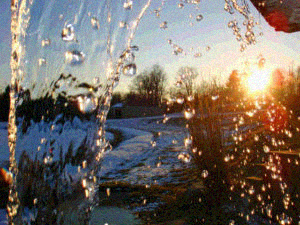# Image Steganography using OpenCV in Python

Image Steganography is the process of hiding secret data in some image. In this post, we will hide one image inside another and convert it into another image and then extract back both the images from the previous image.

The idea behind image-based Steganography is very simple. Images are composed of digital data (pixels), which describes what’s inside the picture, usually the colors of all the pixels. Since we know every image is made up of pixels and every pixel contains 3-values (red, green, blue).

For example, suppose we have to hide img2 in img1, where both img1 and img2 are `numpy nd` array of pixel values. The size of img2 must be less than the size of img1. We are using color images, hence both will have 3 values (red, green, blue). Each pixel value varies from 0 to 255, so each pixel value is of 1 byte or 8 bits. Let `img[i][j][l]` be the pixel value at location `(i, j)` and of channel l where i varies from 0 to width and j varies from 0 to height and l varies from 0 to 2.

Note: The quality of the new images is a little bit less than the old images.

#### Encoding

Let `img1[i][j][l]` and `img2[i][j][l]` be some pixel value of each image. Let v1 be 8 bits binary representation of `img1[i][j][l]` and v2 be 8 bits binary representation of `img2[i][j][l]`. Therefore, `v3=v1[:4]+v2[:4]`, where, v3 is the first 4 bits of v1 and v2. Then we assign `img1[i][j][l]` to v3.

Here img1 is the final image produced after encoding.

#### Decoding

Let `img[i][j][l]` be the pixel value of the image. Let v1 be 8 bits binary representation of `img[i][j][l]`. Let `v2=v1[:4]+4` random bits and `v3=v1[4:]+4` random bits. Then we assign `img1[i][j][l]` to v2 and `img2[i][j][l]` to v3.

Here img1 and img2 are the final images produced after decoding.

Implementation

Lets’ consider the images used are as follows:

Image 1Image 2We want to hide image2 in image1. Below is the implementation.

 `# Python program to demonstrate ` `# image steganography using OpenCV ` ` `  ` `  `import` `cv2 ` `import` `numpy as np ` `import` `random ` ` `  ` `  `# Encryption function ` `def` `encrypt(): ` `     `  `    ``# img1 and img2 are the ` `    ``# two input images ` `    ``img1 ``=` `cv2.imread(``'pic1.jpg'``) ` `    ``img2 ``=` `cv2.imread(``'pic2.jpg'``) ` `     `  `    ``for` `i ``in` `range``(img2.shape[``0``]): ` `        ``for` `j ``in` `range``(img2.shape[``1``]): ` `            ``for` `l ``in` `range``(``3``): ` `                 `  `                ``# v1 and v2 are 8-bit pixel values ` `                ``# of img1 and img2 respectively ` `                ``v1 ``=` `format``(img1[i][j][l], ``'08b'``) ` `                ``v2 ``=` `format``(img2[i][j][l], ``'08b'``) ` `                 `  `                ``# Taking 4 MSBs of each image ` `                ``v3 ``=` `v1[:``4``] ``+` `v2[:``4``]  ` `                 `  `                ``img1[i][j][l]``=` `int``(v3, ``2``) ` `                 `  `    ``cv2.imwrite(``'pic3in2.png'``, img1) ` ` `  `     `  `# Decryption function ` `def` `decrypt(): ` `     `  `    ``# Encrypted image ` `    ``img ``=` `cv2.imread(``'pic3in2.png'``)  ` `    ``width ``=` `img.shape[``0``] ` `    ``height ``=` `img.shape[``1``] ` `     `  `    ``# img1 and img2 are two blank images ` `    ``img1 ``=` `np.zeros((width, height, ``3``), np.uint8) ` `    ``img2 ``=` `np.zeros((width, height, ``3``), np.uint8) ` `     `  `    ``for` `i ``in` `range``(width): ` `        ``for` `j ``in` `range``(height): ` `            ``for` `l ``in` `range``(``3``): ` `                ``v1 ``=` `format``(img[i][j][l], ``'08b'``) ` `                ``v2 ``=` `v1[:``4``] ``+` `chr``(random.randint(``0``, ``1``)``+``48``) ``*` `4` `                ``v3 ``=` `v1[``4``:] ``+` `chr``(random.randint(``0``, ``1``)``+``48``) ``*` `4` `                 `  `                ``# Appending data to img1 and img2 ` `                ``img1[i][j][l]``=` `int``(v2, ``2``) ` `                ``img2[i][j][l]``=` `int``(v3, ``2``) ` `     `  `    ``# These are two images produced from ` `    ``# the encrypted image ` `    ``cv2.imwrite(``'pic2_re.png'``, img1) ` `    ``cv2.imwrite(``'pic3_re.png'``, img2) ` `     `  `     `  `# Driver's code ` `encrypt() ` `decrypt() `

Output:

After Encryption:After Decryption:

Image 1Image 2Attention geek! Strengthen your foundations with the Python Programming Foundation Course and learn the basics.

To begin with, your interview preparations Enhance your Data Structures concepts with the Python DS Course.

My Personal Notes arrow_drop_upCheck out this Author's contributed articles.

If you like GeeksforGeeks and would like to contribute, you can also write an article using contribute.geeksforgeeks.org or mail your article to contribute@geeksforgeeks.org. See your article appearing on the GeeksforGeeks main page and help other Geeks.

Please Improve this article if you find anything incorrect by clicking on the "Improve Article" button below.

Article Tags :

1

Please write to us at contribute@geeksforgeeks.org to report any issue with the above content.